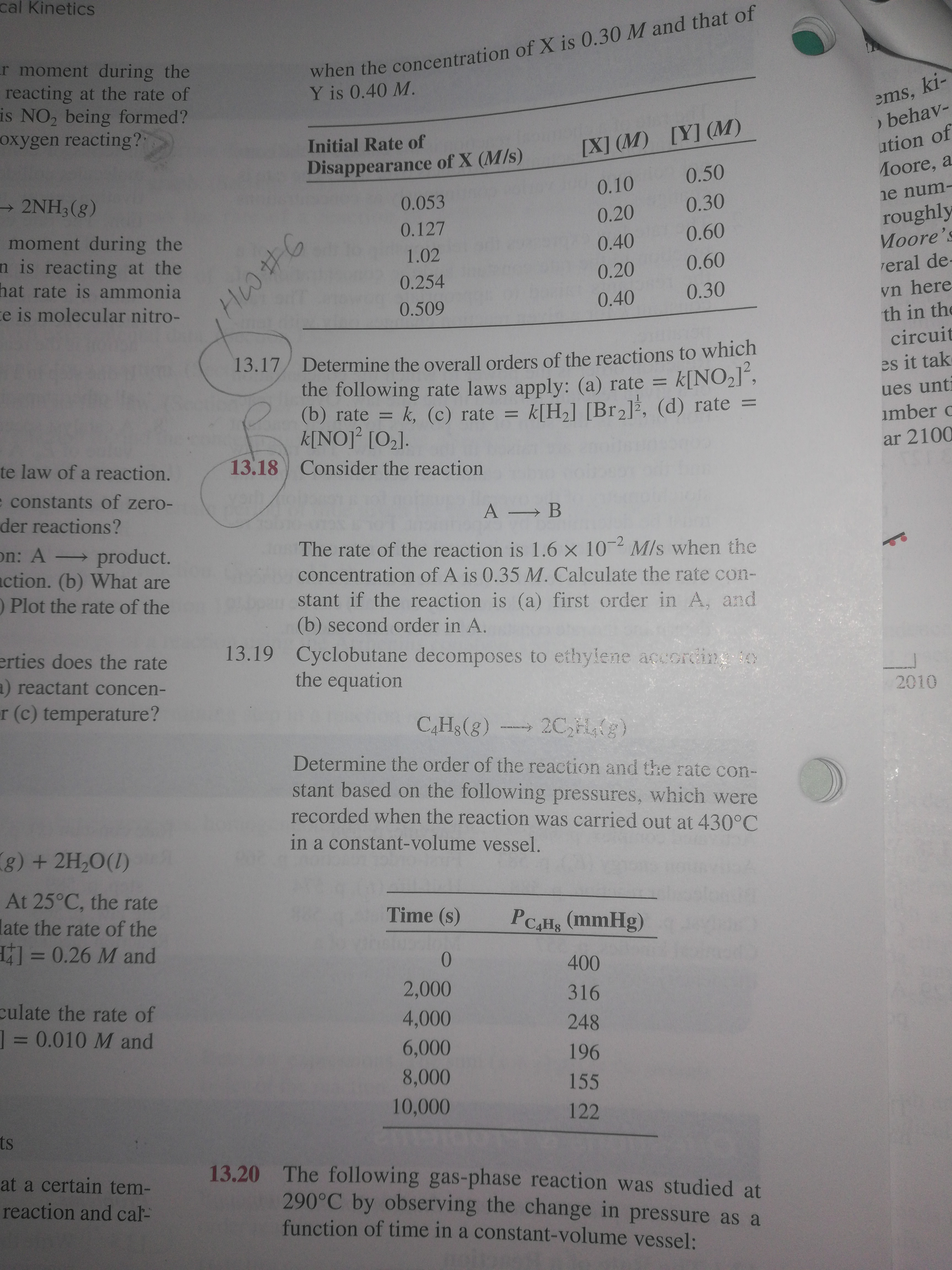# cal Kineticswhen the concentration of X is 0.30 M and that ofY is 0.40 M.r moment during thereacting at the rate ofis NO2 being formed?oxygen reacting?ems, ki-behav-[Y] (M)Initial Rate of[X] (M)ution ofDisappearance of X (M/s)0.50Лoore, a- 2NH3(g)0.100.053ne num-0.300.20roughlyMoore's0.127moment during then is reacting at thehat rate is ammonia0.600.401.020.600.20reral de-0.254vn hereth in the0.30te is molecular nitro-0.400.509circuit13.17 Determine the overall orders of the reactions to whichk[NO,J²,k, (c) rate = k[H2] [Br2]³, (d) ratees it takthe following rate laws apply: (a) rate =ues unti(b) rate =k[NO]° [O2].=imber oar 2100te law of a reaction.13.18Consider the reactione constants of zero-der reactions?A Bon: A product.action. (b) What areO Plot the rate of theThe rate of the reaction is 1.6 x 10 M/s when theconcentration of A is 0.35 M. Calculate the rate con--2stant if the reaction is (a) first order in A, and(b) second order in A.erties does the rate13.19 Cyclobutane decomposes to ethylene according othe equationa) reactant concen-2010r (c) temperature?C,Hs(g)2C,Hg)Determine the order of the reaction and the rate con-stant based on the following pressures, which wererecorded when the reaction was carried out at 430°Cin a constant-volume vessel.g) + 2H,O(1)At 25°C, the ratelate the rate of the330.26 M andTime (s)PCAHs (mmHg)%D4002,000316culate the rate of130.010 M and4,000248%3D6,0001968,00015510,000122ts13.20 The following gas-phase reaction was studied at290°C by observing the change in pressure as afunction of time in a constant-volume vessel:at a certain tem-reaction and cal-

Question
1 views

13.17help_outlineImage Transcriptionclosecal Kinetics when the concentration of X is 0.30 M and that of Y is 0.40 M. r moment during the reacting at the rate of is NO2 being formed? oxygen reacting? ems, ki- behav- [Y] (M) Initial Rate of [X] (M) ution of Disappearance of X (M/s) 0.50 Лoore, a - 2NH3(g) 0.10 0.053 ne num- 0.30 0.20 roughly Moore's 0.127 moment during the n is reacting at the hat rate is ammonia 0.60 0.40 1.02 0.60 0.20 reral de- 0.254 vn here th in the 0.30 te is molecular nitro- 0.40 0.509 circuit 13.17 Determine the overall orders of the reactions to which k[NO,J², k, (c) rate = k[H2] [Br2]³, (d) rate es it tak the following rate laws apply: (a) rate = ues unti (b) rate = k[NO]° [O2]. = imber o ar 2100 te law of a reaction. 13.18 Consider the reaction e constants of zero- der reactions? A B on: A product. action. (b) What are O Plot the rate of the The rate of the reaction is 1.6 x 10 M/s when the concentration of A is 0.35 M. Calculate the rate con- -2 stant if the reaction is (a) first order in A, and (b) second order in A. erties does the rate 13.19 Cyclobutane decomposes to ethylene according o the equation a) reactant concen- 2010 r (c) temperature? C,Hs(g) 2C,Hg) Determine the order of the reaction and the rate con- stant based on the following pressures, which were recorded when the reaction was carried out at 430°C in a constant-volume vessel. g) + 2H,O(1) At 25°C, the rate late the rate of the 330.26 M and Time (s) PCAHs (mmHg) %D 400 2,000 316 culate the rate of 130.010 M and 4,000 248 %3D 6,000 196 8,000 155 10,000 122 ts 13.20 The following gas-phase reaction was studied at 290°C by observing the change in pressure as a function of time in a constant-volume vessel: at a certain tem- reaction and cal- fullscreen
check_circle

Step 1

The order of each reactant in a reaction is represented by the exponential term of the respective reactant present in the rate law and the overall order of th...

### Want to see the full answer?

See Solution

#### Want to see this answer and more?

Solutions are written by subject experts who are available 24/7. Questions are typically answered within 1 hour.*

See Solution
*Response times may vary by subject and question.
Tagged in